# Add and Subtract Fractions with Unlike Denominators Lesson Plan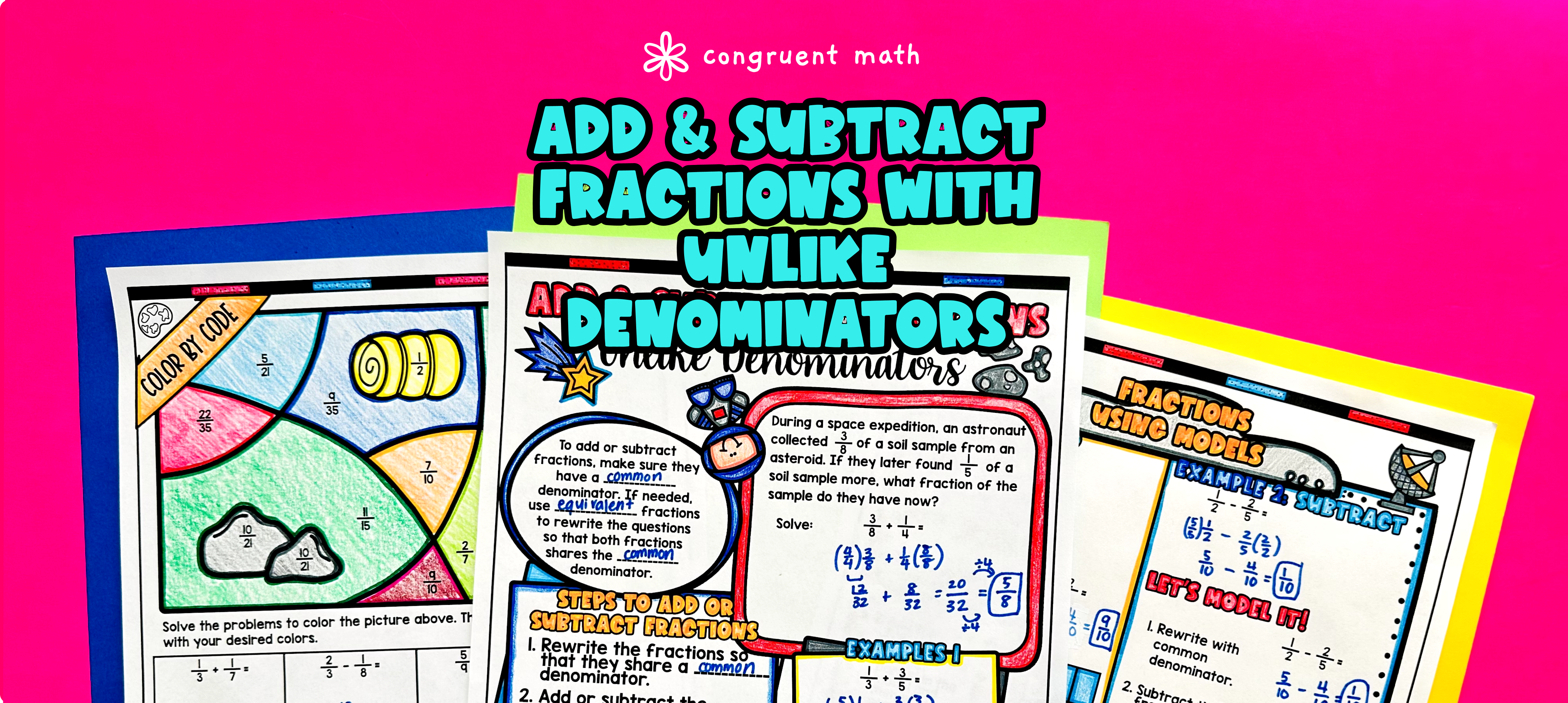Pin This

## Overview

Ever wondered how to teach adding and subtracting fractions with unlike denominators in an engaging way to your 5th or 6th-grade students?

In this lesson plan, students will learn about fraction addition and subtraction and their real-life applications. Through artistic, interactive guided notes, check for understanding, a practice activity that includes a doodle & color by number worksheet, and a maze worksheet, students will gain a comprehensive understanding of adding and subtracting fractions with unlike denominators.

The lesson culminates with a real-life example that explores how this math skill can be applied in practical situations. Students will read and write about real-life uses of adding and subtracting fractions.

## Get the Lesson Materials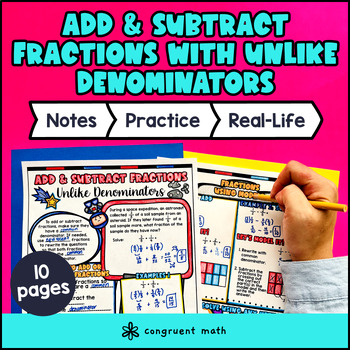\$4.25

## Learning Objectives

After this lesson, students will be able to:

• Add and subtract fractions with unlike denominators

• Use visual aids and doodles to enhance understanding of fraction addition and subtraction

• Explain real life applications of fractions in real life

## Prerequisites

Before this lesson, students should be familiar with:

• Basic addition and subtraction skills of whole numbers

• Understand how to generate equivalent fractions

• Knowledge of how to find common multiples and factors

## Key Vocabulary

• Unlike Denominators

• Common Denominator

• Visual Models

## Procedure

### Introduction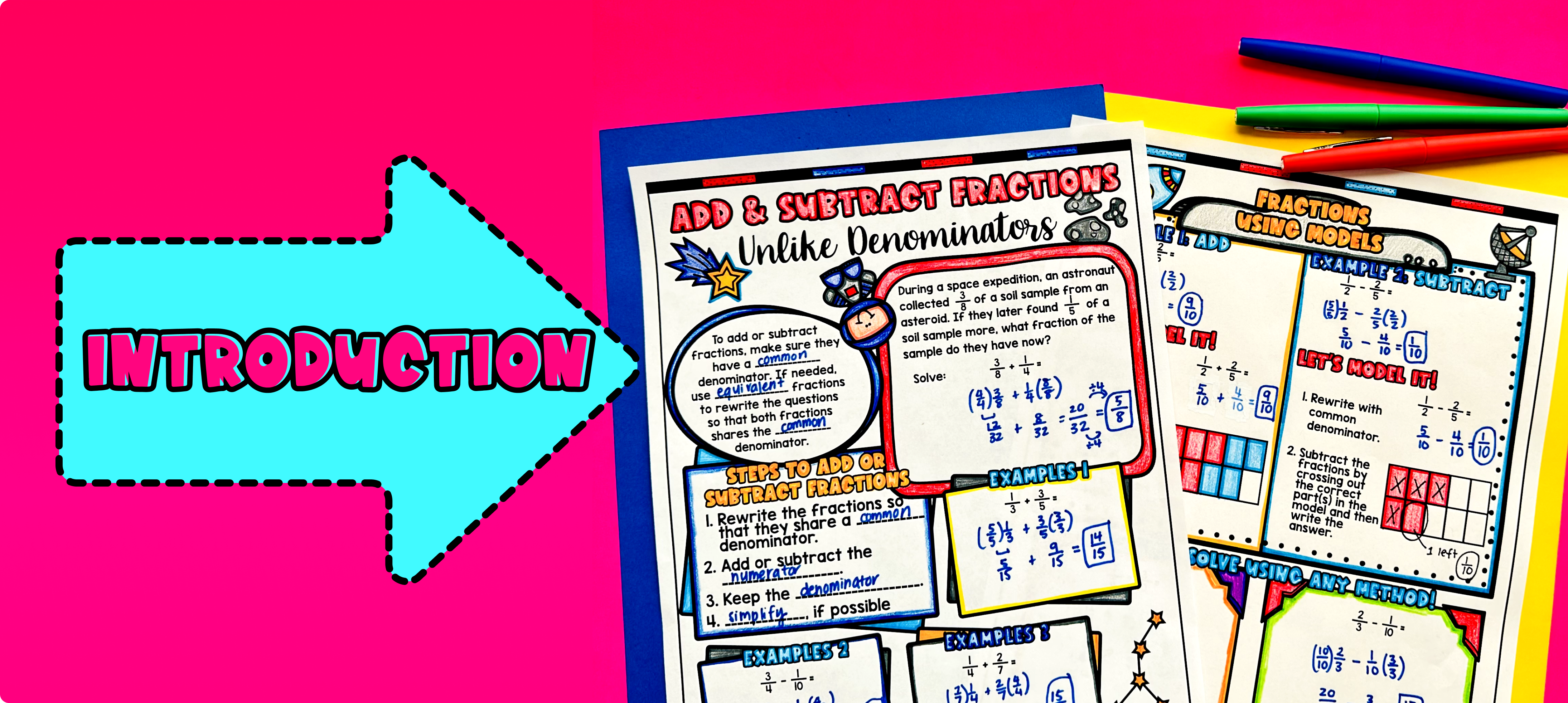Pin This

As a hook, ask students why it is important to know how to add and subtract fractions with unlike denominators in real-life situations.

Use the first page of the guided notes to introduce the concept of adding and subtracting fractions with unlike denominators. Walk through the steps of finding a common denominator, adding or subtracting the numerators, and simplifying the fraction. Emphasize the importance of finding a common denominator to make the fractions compatible.

Use the second page of the guided notes to introduce how to use visual models to solve fractions addition and subtracting with unlike denominators. Walk through the steps of finding a common denominator, subtracting the numerators, and simplifying the fraction.

Based on student responses, reteach concepts that students need extra help with. If your class has a wide range of proficiency levels, you can pull out students for reteaching, and have more advanced students begin work on the practice exercises.

### Practice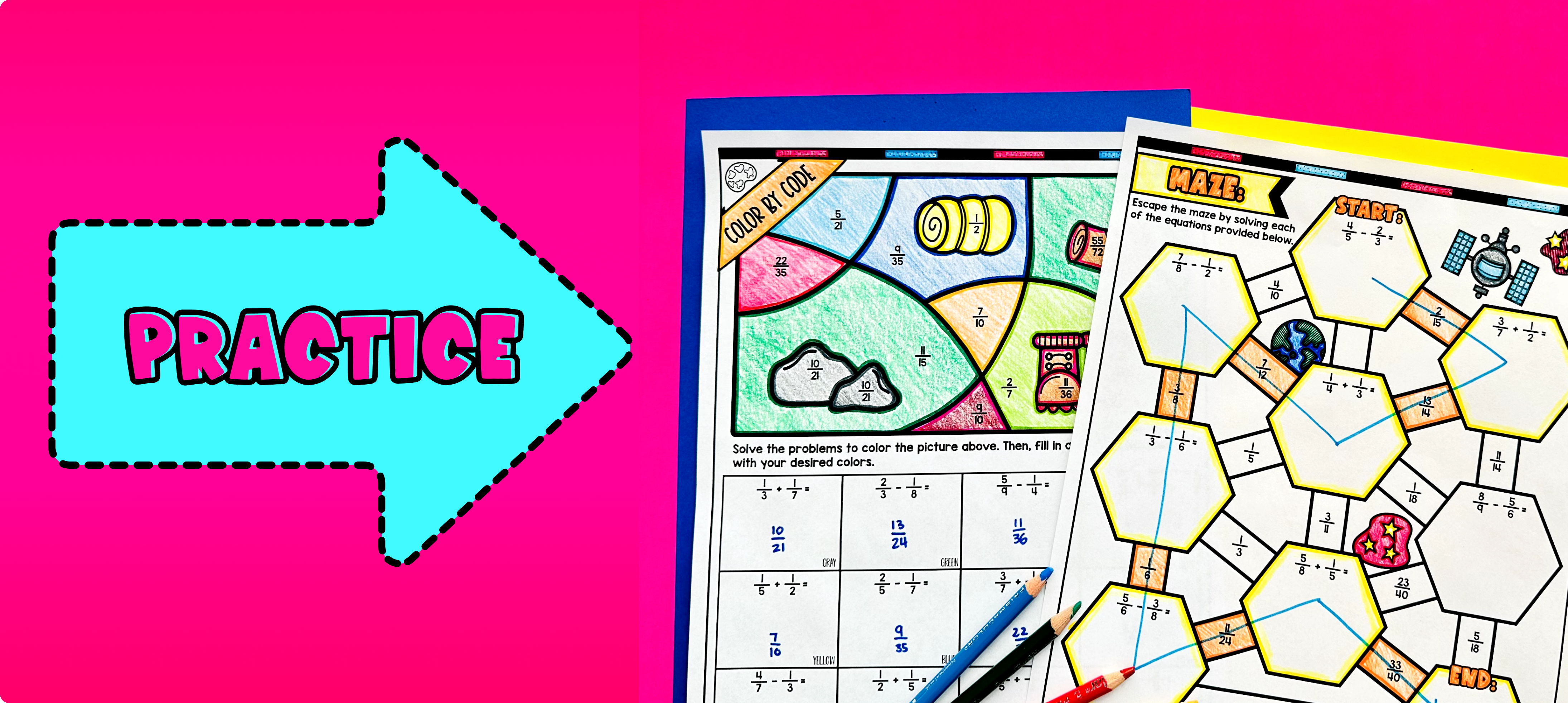Pin This

Have students practice adding and subtracting fractions with unlike denominators using the practice worksheet provided in the resource in the first two pages of the guided notes. Walk around the classroom to answer any questions students may have.

Fast finishers can continue practicing with the maze activities (page 3) or color by number (page 4) included in the resource. You can assign these activities as extra practice or as homework for the remainder of the class.

### Real-Life Application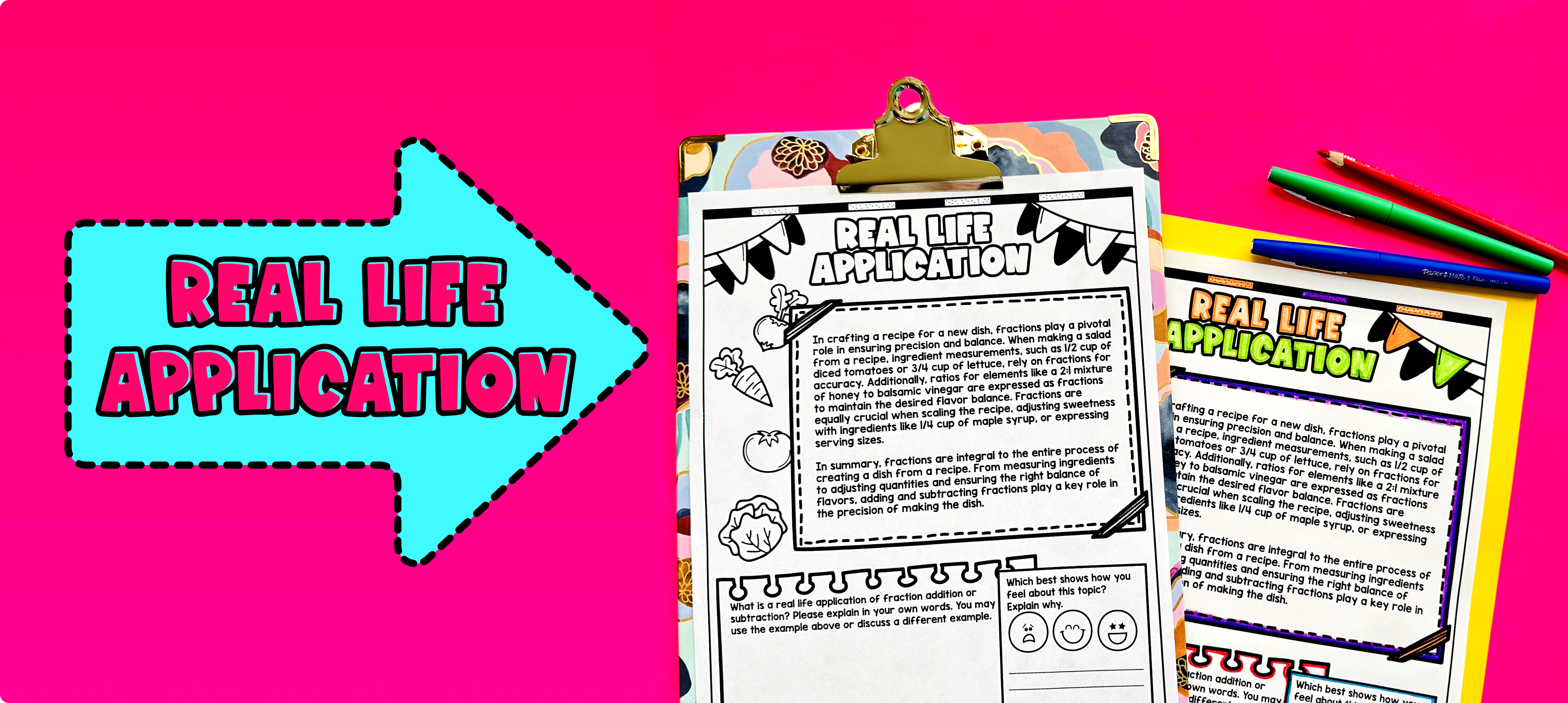Pin This

Use the last page of the guided notes to bring the class back together, and introduce the concept of real-life application of adding and subtracting fractions with unlike denominators. Explain to the students that understanding how to add and subtract fractions is important in many real-life situations.

Some examples of real-life applications of adding and subtracting fractions include:

1. Recipes: Fractions are commonly used in cooking and baking. When following a recipe, you often have to adjust the measurements based on the number of servings or the size of the dish. Adding or subtracting fractions allows you to adjust the quantities of ingredients accordingly.

2. Measurements: Fractions are also used in various measurements, such as measuring ingredients for a recipe or calculating distances. For example, if you want to measure a distance that is less than a whole unit, you may need to add or subtract fractions to get an accurate measurement.

Discuss these examples with the students and encourage them to brainstorm other situations where adding and subtracting fractions might be useful in their daily lives. This discussion will help them see the relevance and practical application of the skills they are learning.

## Extensions

If you’re looking for digital practice for adding and subtracting fractions with unlike denominators, try my Pixel Art activities in Google Sheets. Every answer is automatically checked, and correct answers unlock parts of a mystery picture. It’s incredibly fun, and a powerful tool for differentiation.

Here are some activities to explore: